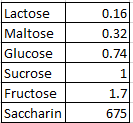### CAT 1999 Question Paper Question 27

Instructions

DIRECTIONS for the following two questions: The following table presents the sweetness of different items relative to sucrose, whose sweetness is taken to be 1.00.Question 27

# Approximately how many times sweeter than sucrose is a mixture consisting of glucose, sucrose and fructose in the ratio of 1: 2: 3?

Solution

The relative sweetness of the mixture is (1*0.74 + 2*1 + 3*1.7) / (1+2+3) = 7.84/6 = 1.30

Option a) is the correct answer.

### View Video Solution

• All Quant CAT Formulas and shortcuts PDF
• 30+ CAT previous papers with solutions PDF